Скачать презентацию Form of a linear equation written as y

34c59df28d2a7fbb583b59f522531e5e.ppt

• Количество слайдов: 10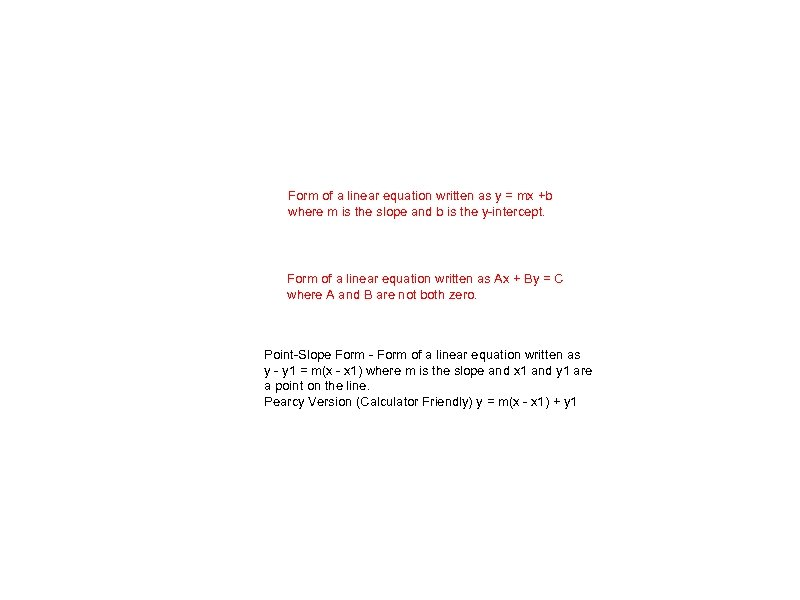Form of a linear equation written as y = mx +b where m is the slope and b is the y-intercept. Form of a linear equation written as Ax + By = C where A and B are not both zero. Point-Slope Form - Form of a linear equation written as y - y 1 = m(x - x 1) where m is the slope and x 1 and y 1 are a point on the line. Pearcy Version (Calculator Friendly) y = m(x - x 1) + y 1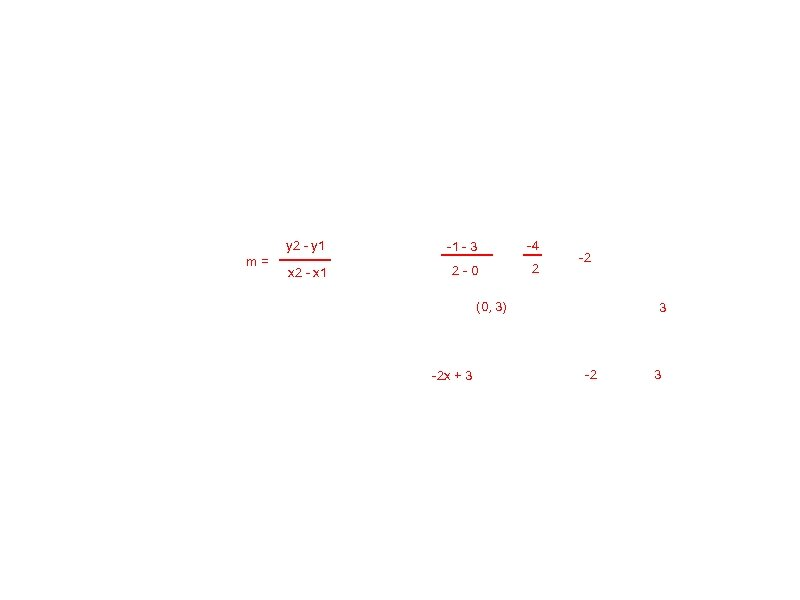y 2 - y 1 m= -1 - 3 -4 x 2 - x 1 2 -0 2 -2 (0, 3) -2 x + 3 3 -2 3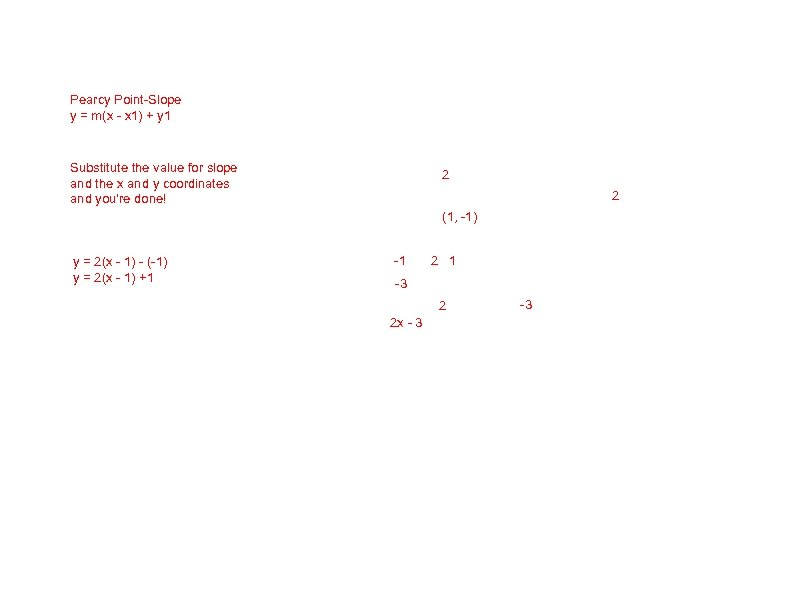Pearcy Point-Slope y = m(x - x 1) + y 1 Substitute the value for slope and the x and y coordinates and you're done! 2 2 (1, -1) y = 2(x - 1) - (-1) y = 2(x - 1) +1 -1 2 1 -3 2 2 x - 3 -3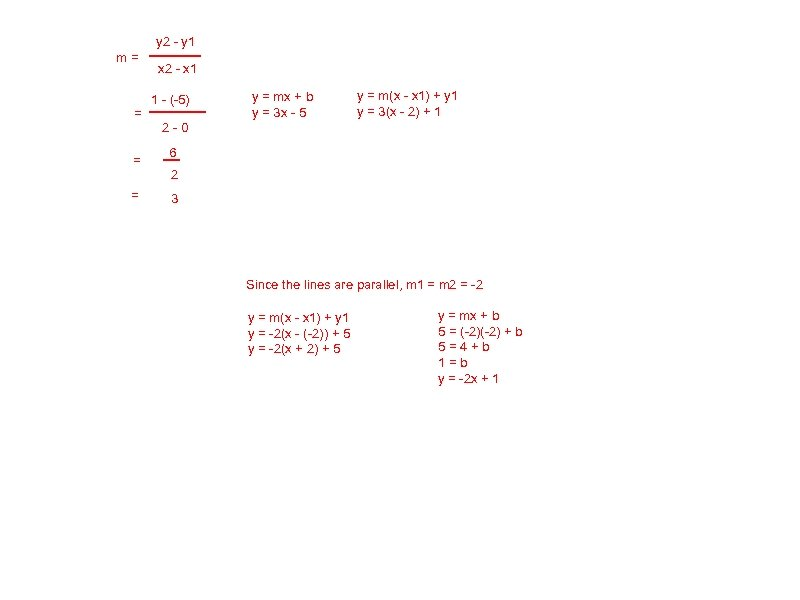y 2 - y 1 m= = x 2 - x 1 1 - (-5) y = mx + b y = 3 x - 5 y = m(x - x 1) + y 1 y = 3(x - 2) + 1 2 -0 6 2 3 Since the lines are parallel, m 1 = m 2 = -2 y = m(x - x 1) + y 1 y = -2(x - (-2)) + 5 y = -2(x + 2) + 5 y = mx + b 5 = (-2) + b 5=4+b 1=b y = -2 x + 1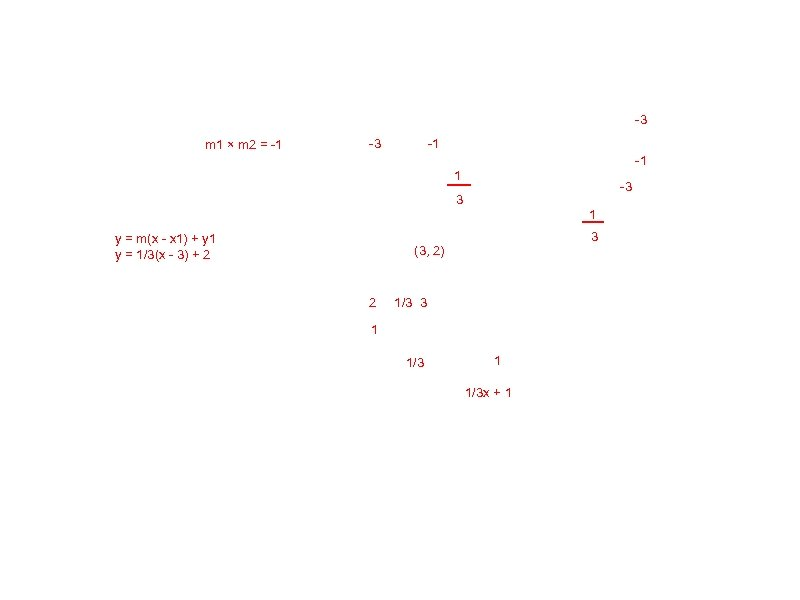-3 m 1 × m 2 = -1 -3 -1 -1 1 -3 3 1 y = m(x - x 1) + y 1 y = 1/3(x - 3) + 2 3 (3, 2) 2 1/3 3 1 1/3 x + 1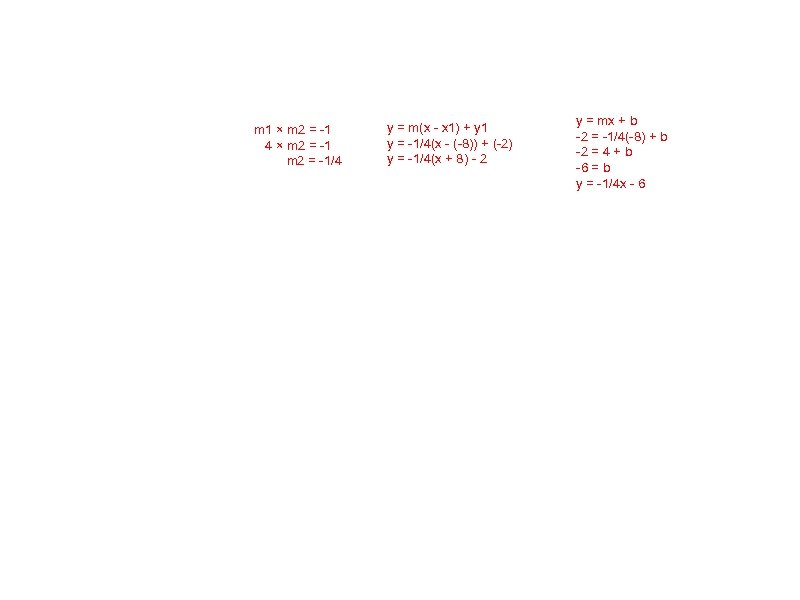m 1 × m 2 = -1 4 × m 2 = -1/4 y = m(x - x 1) + y 1 y = -1/4(x - (-8)) + (-2) y = -1/4(x + 8) - 2 y = mx + b -2 = -1/4(-8) + b -2 = 4 + b -6 = b y = -1/4 x - 6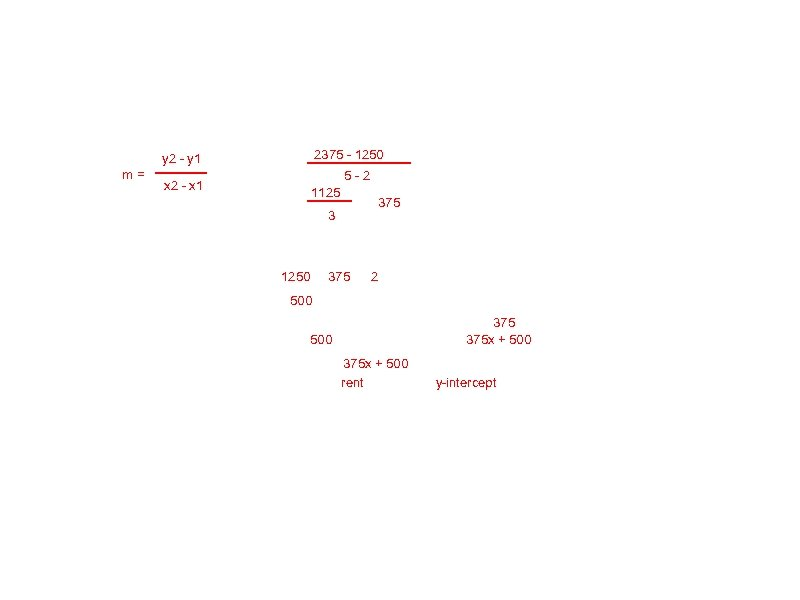2375 - 1250 y 2 - y 1 m= 5 -2 x 2 - x 1 1125 375 3 1250 375 2 500 375 x + 500 rent y-intercept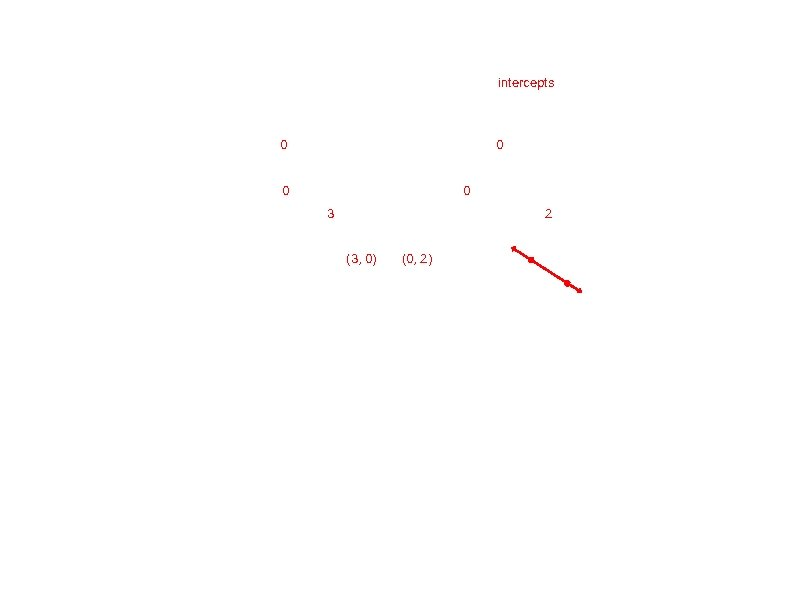intercepts 0 0 3 2 (3, 0) (0, 2)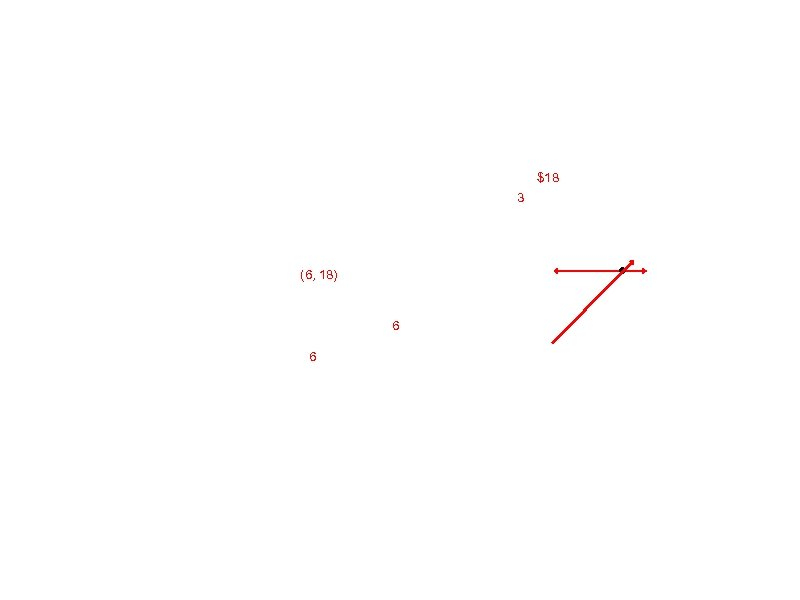\$18 3 (6, 18) 6 6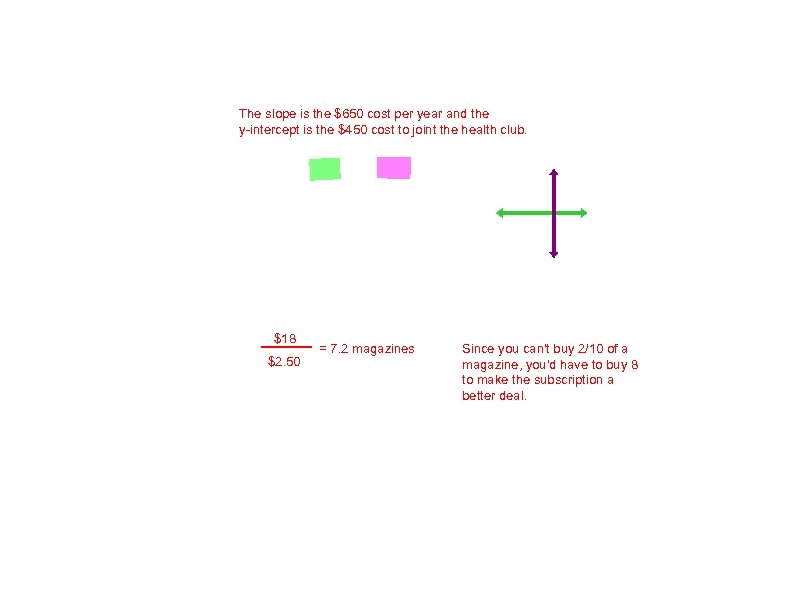The slope is the \$650 cost per year and the y-intercept is the \$450 cost to joint the health club. \$18 \$2. 50 = 7. 2 magazines Since you can't buy 2/10 of a magazine, you'd have to buy 8 to make the subscription a better deal.